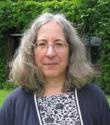Plenary SpeakersMark J. Ablowitz

Title: The Nonlinear Waves—Always Intriguing

Abstract: The study of nonlinear waves is filled with remarkable discoveries, one of them being `solitons’. Some history, background, and applications in water waves and nonlinear optics, specifically `optical graphene’, will be discussed.Susanne Brenner
Louisiana State University

Title: Finite Element Methods for Fourth Order Elliptic Variational Inequalities

Abstract: Fourth order elliptic variational inequalities appear in obstacle problems for Kirchhoff plates and optimal control problems constrained by second order elliptic partial differential equations. The numerical analysis of these variational inequalities is more challenging than the analysis in the second order case because the complementarity forms of fourth order variational inequalities only exist in a weak sense.  In this talk we will present a new approach to the analysis of finite element methods for fourth order elliptic variational inequalities that are applicable to C1 finite element methods, classical nonconforming finite element methods, and discontinuous Galerkin methods.Arnd Scheel
University of Minnesota

Title: Coherent structures in nonlocal equations

Abstract: I'll present recent work on pulses and fronts in systems with nonlocal coupling. I'll first discuss pinning and unpinning of fronts. Near the Maxwell point, that is, when potential energies of the asymptotic equilibria are close, interfaces are often discontinuous and cannot propagate: they are pinned. I'll describe results that characterize pinning regions in parameter space and and show that speeds obey an unusual but universal mu^3/2 asymptotic which is different from conventional mu^1/2 asymptotics in discrete systems. I'll also give some motivation and speculation how speed asymptotics may depend in a universal fashion on kernel regularity properties.

In the second part of the talk, I'll explore some of the techniques involved in the study of such traveling wave problems. In particular, I'll explain how ``spatial dynamics'' can be ``translated'' to traveling-wave problems that cannot be cast as differential equations in a spatial variable. As an application, I'll describe the construction of an excitation pulse in a nonlocal FitzHugh-Nagumo equation.Junping Wang
National Science Foundation

Title: Weak Galerkin Finite Element Methods for PDEs

Abstract:  This talk shall introduce a new numerical technique, called weak Galerkin finite element method (WG), for partial differential equations. The presentation will start with the second order elliptic equation, for which WG shall be applied and explained in detail. In particular, the concept of weak gradient will be introduced and discussed for its role in the design of weak Galerkin finite element schemes. The speaker will then introduce a general notion of weak differential operators, such as weak Hessian, weak divergence, and weak curl etc. These weak differential operators shall serve as building blocks for WG finite element methods for other class of partial differential equations, such as the Stokes equation, the biharmonic equation, the Maxwell equations in electron magnetics theory, and div-curl systems. The speaker will demonstrate how WG can be applied to each of the applications, with a discussion on the main features. Furthermore, a mathematical convergence theory shall be briefly given for some applications. The talk should be accessible to graduate students with adequate training in computational methods.

MISSOURI UNIVERSITY OF SCIENCE AND TECHNOLOGY             Founded 1870  |  Rolla, Missouri